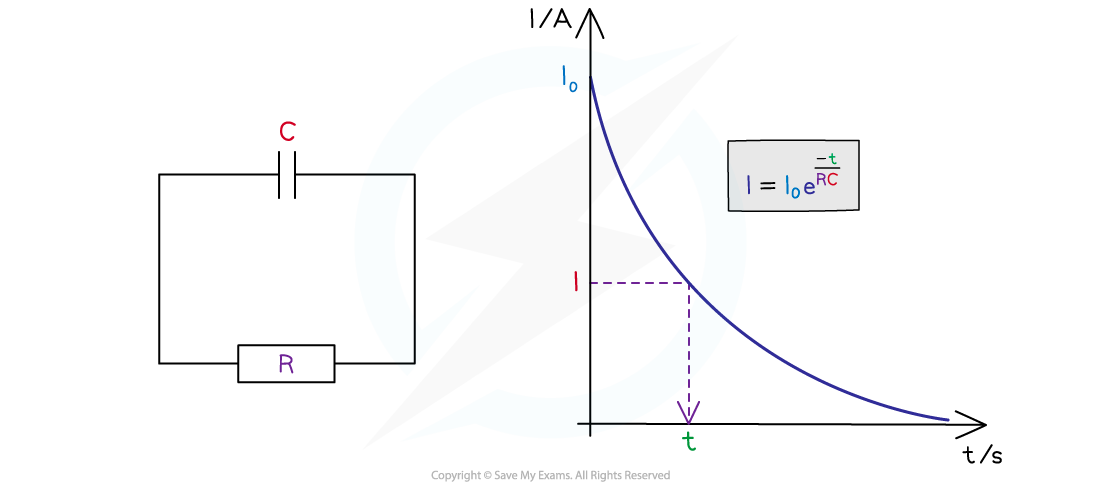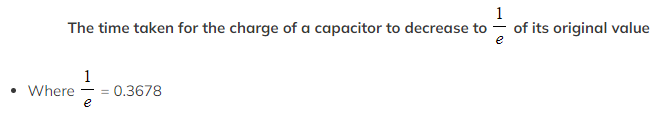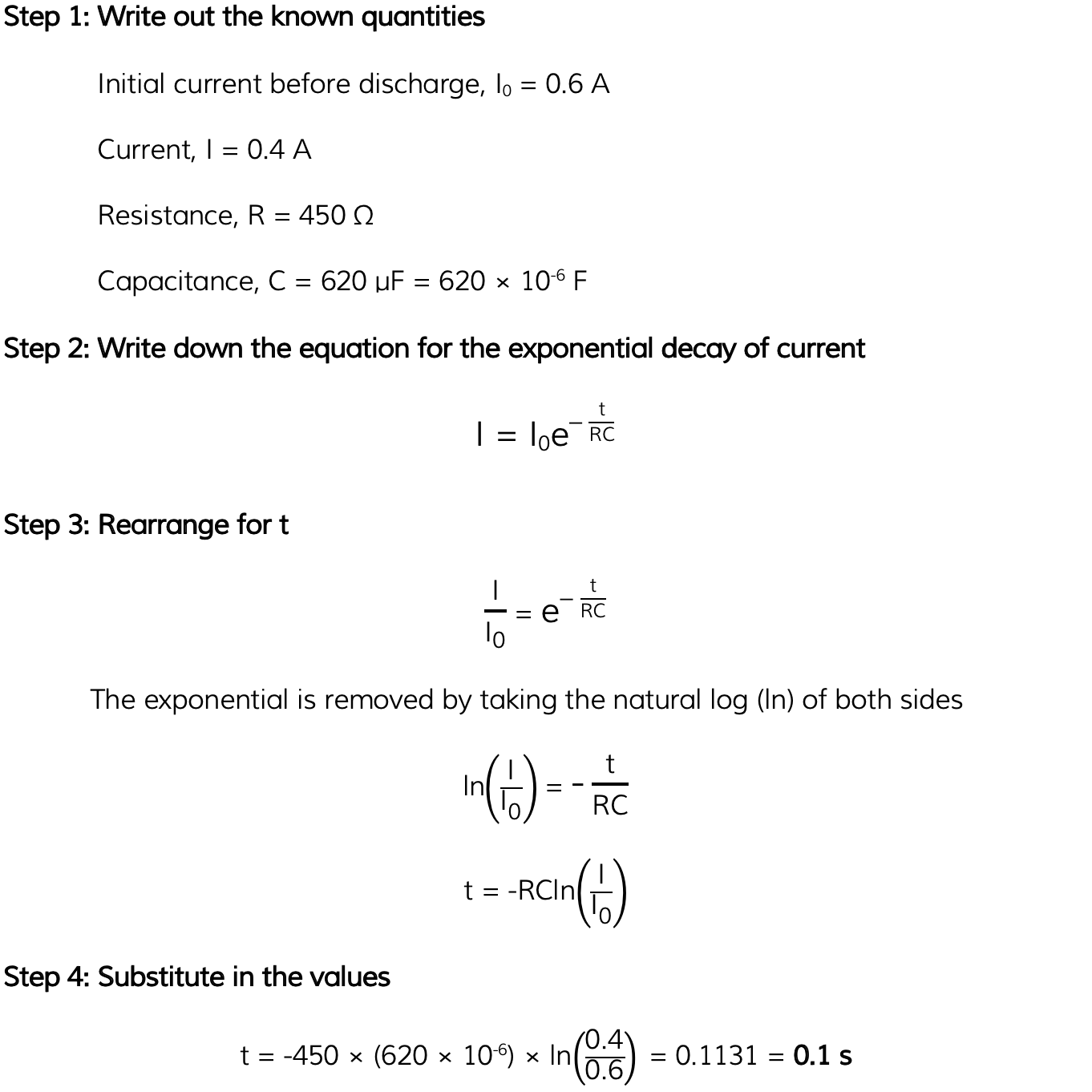# IB DP Physics: HL复习笔记11.3.7 Discharge Calculations

### Capacitor Discharge Equation

• The time constant is used in the exponential decay equations for the current, charge or potential difference (p.d) for a capacitor discharging through a resistor
• These can be used to determine the amount of current, charge or p.d left after a certain amount of time for a discharging capacitor
• This exponential decay means that no matter how much charge is initially on the plates, the amount of time it takes for that charge to halve is the same
• The exponential decay of current on a discharging capacitor is defined by the equation:• Where:
• I = current (A)
• I0 = initial current before discharge (A)
• e = the exponential function
• t = time (s)
• RC = resistance (Ω) × capacitance (F) = the time constant τ (s)
• This equation shows that the smaller the time constant τ, the quicker the exponential decay of the current when discharging
• Also, how big the initial current is affects the rate of discharge
• If I0 is large, the capacitor will take longer to discharge
• Note: during capacitor discharge, I0 is always larger than I, as the current I will always be decreasingValues of the capacitor discharge equation on a graph and circuit

• The current at any time is directly proportional to the p.d across the capacitor and the charge across the parallel plates
• Therefore, this equation also describes the charge on the capacitor after a certain amount of time:• Where:
• Q = charge on the capacitor plates (C)
• Q0 = initial charge on the capacitor plates (C)
• As well as the p.d after a certain amount of time:• Where:
• V = p.d across the capacitor (C)
• V0 = initial p.d across the capacitor (C)

#### The Exponential Function e

• The symbol e represents the exponential constant, a number which is approximately equal to e = 2.718...
• On a calculator, it is shown by the button ex
• The inverse function of ex is ln(y), known as the natural logarithmic function
• This is because, if ex = y, then x = ln (y)
• The 0.37 in the definition of the time constant arises as a result of the exponential constant, the true definition is:Worked Example

The initial current through a circuit with a capacitor of 620 µF is 0.6 A. The capacitor is connected across the terminals of a 450 Ω resistor.

Calculate the time taken for the current to fall to 0.4 A.